﻿ 基于NI Vision Assistant的樱桃缺陷检测方法 Detection of Cherry Defects Based on NI Vision Assistant

Computer Science and Application
Vol.07 No.12(2017), Article ID:22922,11 pages
10.12677/CSA.2017.712131

Detection of Cherry Defects Based on NI Vision Assistant

Zhao Wang1, Pengle Cheng1, Yilong Huangpu2, Hongtao Gu1, Jingshan Zhang2

1Beijing Forestry University, Beijing

2Beijing Wuzi University, BeijingReceived: Nov. 15th, 2017; accepted: Nov. 28th, 2017; published: Dec. 5th, 2017ABSTRACT

In order to improve the status of cherry sorting in China, the automatic screening of cherry fruit defects and the improvement of cherry production efficiency were mainly carried out. Combined with the existing image processing methods, this paper designed a set of cherry defect detection device based on visual processing software NI Vision Assistant. In this experiment, using image acquisition system to obtain the image and then the algorithm to extract the cherry fruit defect part; finally the defect location information is sent to the screening mechanism, so as to complete the detection and screening of defects of cherry. Finally, the experimental results show that the screening method is fast; the experimental instruments and methods are relatively simple; and the comprehensive detection accuracy is relatively high, which basically meets the requirements of the detection of defective cherries in actual production.

Keywords:Machine Vision, Image Processing, Defect Detection, Cherries

1北京林业大学，北京

2北京物资学院，北京1. 引言

2. 缺陷检测视觉系统方案概述

2.1. 实验材料

2.2. 视觉系统示意图

2.3. 视觉系统硬件选择

2.4. 系统工作过程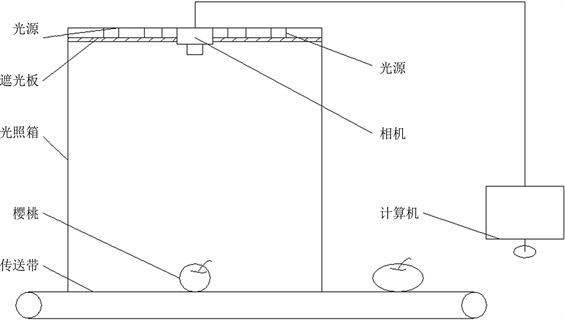Figure 1. Sketch map of visual system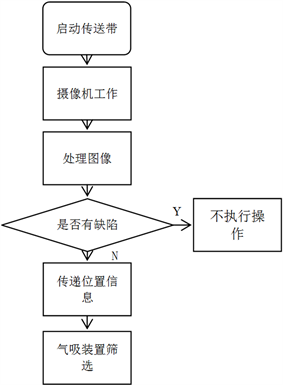Figure 2. System work flow chart

3. 图像特征提取及缺陷检测算法

3.1. 去背景及图像分割

3.2. 涉及算法或函数

3.2.1. 阈值分割算法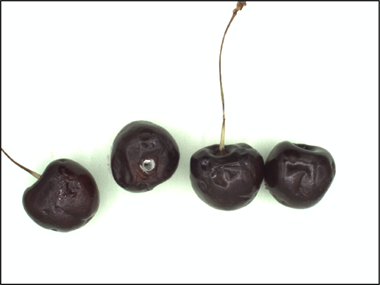Figure 3. A color chart of several cherries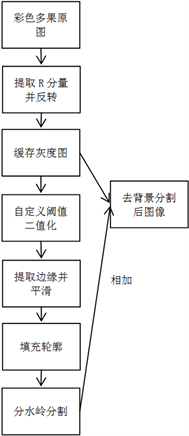Figure 4. Flow chart of individual fruit image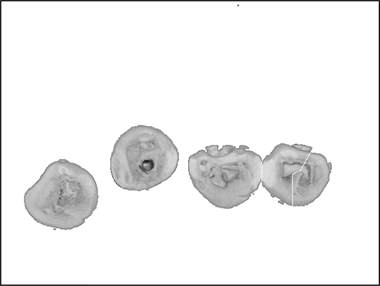Figure 5. Removing background segmentation

${\rho }_{1}=\frac{{A}_{1}}{A×B}$ (1)

${\rho }_{2}=\frac{{A}_{2}}{A×B}$ (2)

${A}_{1}+{A}_{2}=A×B$ (3)

${\rho }_{1}×{\rho }_{2}=1$ (4)

$N={N}_{1}×{P}_{1}+{N}_{2}×{P}_{2}$ (5)

$P={\rho }_{1}×{\left(N-{N}_{1}\right)}^{2}+{\rho }_{2}×{\left(N-{N}_{2}\right)}^{2}$ (6)

$P={\rho }_{1}×{\rho }_{2}×{\left({N}_{1}×{N}_{2}\right)}^{2}$ (7)

3.2.2. 边缘检测算子

Laplacian算子

Laplacian算子原理是对于大部分图像而言，其边缘会出现阶跃，则其二阶导数在边缘点两侧异号。其定义式为：

${\nabla }^{2}f\left(x,y\right)=\frac{{\partial }^{2}}{\partial {x}^{2}}f\left(x,y\right)+\frac{{\partial }^{2}}{\partial {y}^{2}}f\left(x,y\right)$ (8)

${\Delta }^{2}f\left(x,y\right)=f\left(x+1,y\right)+f\left(x-1,y\right)+f\left(x,y+1\right)+f\left(x,y-1\right)-4f\left(x,y\right)$ (9)

$\begin{array}{c}{\Delta }^{2}f\left(x,y\right)=f\left(x-1,y-1\right)+f\left(x,y-1\right)+f\left(x+1,y-1\right)+f\left(x-1,y\right)+f\left(x+1,y\right)\\ \text{\hspace{0.17em}}\text{\hspace{0.17em}}+f\left(x-1,y+1\right)+f\left(x,y+1\right)+f\left(x+1,y+1\right)-8f\left(x,y\right)\end{array}$ (10)

Laplacian算子模版如图6所示，基本特点为中心位置系数为正其余系数为负或零，且正负和为0。Laplacian算子对边缘端点及孤立点识别效果显著，这也使得其对噪声非常敏感，所以很少用直接用Laplacian算子进行检测。

Sobel算子

Sobel算子是基于函数卷积来进行图像分析的，具体运算过程就是将原图像函数先进行加权平均而后求其微分，其定义式为：

${s}_{\left(x,y\right)}=MAX\left[{\sum }_{m=1}^{M}{\sum }_{n=1}^{N}f\left(m,n\right){g}_{1,2}\left(i-m,j-n\right)\right]$ (11)

$\begin{array}{c}{\Delta }_{x}{f}_{\left(x,y\right)}=\left[f\left(x-1,y+1\right)+2f\left(x,y+1\right)+f\left(x+1,y+1\right)\right]\\ \text{\hspace{0.17em}}\text{\hspace{0.17em}}-\left[f\left(x-1,y-1\right)+2\left(x,y-1\right)+f\left(x+1,y-1\right)\right]\end{array}$ (12)

$\begin{array}{c}{\Delta }_{y}{f}_{\left(x,y\right)}=\left[f\left(x-1,y-1\right)+2f\left(x-1,y\right)+f\left(x-1,y+1\right)\right]\\ \text{\hspace{0.17em}}\text{\hspace{0.17em}}-\left[f\left(x+1,y-1\right)+2\left(x+1,y\right)+f\left(x+1,y+1\right)\right]\end{array}$ (13)

Sobel算子模版如图7所示，左边表格用于检测水平方向边缘，右边表格用于检测垂直方向的边缘。

Candy算子

Candy算子不同于前面2种算子，其为了避免噪声干扰，选取高斯函数的一阶导函数作为边边缘检测算子。

$G\left(x,y\right)=\frac{1}{2\text{π}{\sigma }^{2}}\mathrm{exp}\left(-\frac{{x}^{2}+{y}^{2}}{2{\sigma }^{2}}\right)$ (14)

$\frac{\partial G\left(x,y\right)}{\partial x}=kx\mathrm{exp}\left(-\frac{{x}^{2}}{2{\sigma }^{2}}\right)\mathrm{exp}\left(-\frac{{y}^{2}}{2{\sigma }^{2}}\right)={h}_{1}\left(x\right){h}_{2}\left(y\right)$ (15)

$\frac{\partial G\left(x,y\right)}{\partial y}=ky\mathrm{exp}\left(-\frac{{y}^{2}}{2{\sigma }^{2}}\right)\mathrm{exp}\left(-\frac{{x}^{2}}{2{\sigma }^{2}}\right)={h}_{1}\left(y\right){h}_{2}\left(x\right)$ (16)

${E}_{x}=\frac{\partial G\left(x,y\right)}{\partial x}\ast f;\text{\hspace{0.17em}}\text{\hspace{0.17em}}{E}_{y}=\frac{\partial G\left(x,y\right)}{\partial y}\ast f$ (17)

$A\left(i,j\right)=\sqrt{{E}_{x}^{2}+{E}_{y}^{2}};\text{\hspace{0.17em}}\text{\hspace{0.17em}}a\left(i,j\right)=\mathrm{arctan}\frac{{E}_{y}\left(i\cdot j\right)}{{E}_{x}\left(i\cdot j\right)}$ (18)Figure 6. Laplacian operator template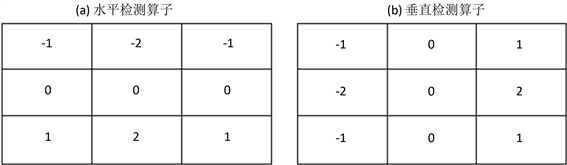Figure 7. Sobel operator template

3.2.3. 滤波处理

3.3. 水果缺陷提取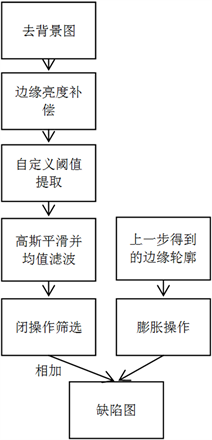Figure 8. Flow chart of defect segmentation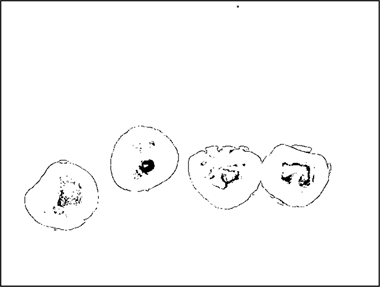Figure 9. Edge compensation and threshold segmentation

3.4. 缺陷樱桃筛选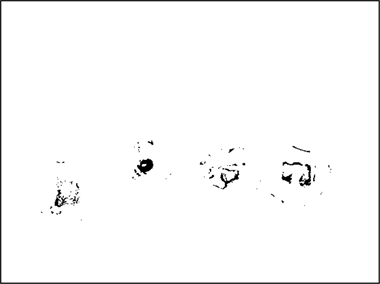Figure 10. Final defect picture

4. 实验数据

· 樱桃在进入光照箱前，若不进行清洗，则有可能存在泥土鸟粪等污垢，而在检测时依然会将它们识别为缺陷，但是如果进行水洗又会降低樱桃的贮藏期限。

· 有些樱桃在采摘过程中果梗会脱落从而露出一个圆形的“缺口”，如果该“缺口”恰好在正上方时，也会被错误的认定为缺陷。

· 虽然设置了自定义阈值，但是由于樱桃缺陷种类众多，且不同种缺陷的表现形式如颜色、亮度、对比度等会有较大差别，因此不存在能将所有缺陷一次性检测出来的方法。

5. 总结

Detection of Cherry Defects Based on NI Vision Assistant[J]. 计算机科学与应用, 2017, 07(12): 1163-1173. http://dx.doi.org/10.12677/CSA.2017.712131

1. 1. Tao, Y., Heinemann, P.H., et al. (1995) Machine Vision for Color Inspection of Potatoes and Apples. Transactions of the ASAE, 38, 1555-1561. https://doi.org/10.13031/2013.27982

2. 2. 应义斌, 景寒松. 黄花梨果形的机器视觉识别方法研究[J]. 农业工程学报, 1999, 15(1): 192-196.

3. 3. 刘佳男. 基于机器视觉的水果表面缺陷识别方法的研究[D]: [硕士学位论文]. 无锡: 江南大学, 2012.

4. 4. 王福杰. 运动水果的形状描述方法与在线检测技术[D]: [博士学位论文]. 杭州: 浙江大学, 2013.

5. 5. 饶秀勤, 应义斌. 基于机器视觉的水果尺寸检测误差分析[J]. 农业工程学报, 2003, 19(1): 121-123.

6. 6. 白菲. 基于机器视觉的柑橘水果外形识别方法研究[D]: [硕士学位论文]. 北京: 中国农业大学, 2005.